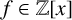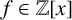Hostname: page-component-cd4964975-4wks4 Total loading time: 0 Render date: 2023-03-30T08:45:50.882Z Has data issue: true Feature Flags: { "useRatesEcommerce": false } hasContentIssue true

## Abstract

We obtain an effective analytic formula, with explicit constants, for the number of distinct irreducible factors of a polynomial$f \in \mathbb {Z}[x]$ . We use an explicit version of Mertens’ theorem for number fields to estimate a related sum over rational primes. For a given$f \in \mathbb {Z}[x]$ , our result yields a finite list of primes that certifies the number of distinct irreducible factors of f.

## MSC classification

Type
Research Article
Information
Journal of the Australian Mathematical Society , December 2022 , pp. 339 - 356
© The Author(s), 2021. Published by Cambridge University Press on behalf of Australian Mathematical Publishing Association Inc.

## Access options

Get access to the full version of this content by using one of the access options below. (Log in options will check for institutional or personal access. Content may require purchase if you do not have access.)

## Footnotes

Communicated by Michael Coons

SRG was supported by NSF grants DMS-2054002 and DMS-1800123.

## References

Bantle, G., ‘On the$A$ -dependence of the linear sieve with application to polynomial sequences’, J. Number Theory 24(2) (1986), 134153.CrossRefGoogle Scholar
Broadbent, S., Kadiri, H., Lumley, A., Ng, N. and Wilk, K., ‘Sharper bounds for the Chebyshev function$\ \theta (x)$ ’, Math. Comp. 90(331) (2021), 22812315.CrossRefGoogle Scholar
Cox, D. A., Little, J. and O’Shea, D., Ideals, Varieties, and Algorithms: An Introduction to Computational Algebraic Geometry and Commutative Algebra, Undergraduate Texts in Mathematics, 4th edn (Springer, Cham, 2015).CrossRefGoogle Scholar
Diamond, H. G. and Halberstam, H., A Higher-Dimensional Sieve Method, Cambridge Tracts in Mathematics, 177 (Cambridge University Press, Cambridge, 2008), with an appendix ‘Procedures for computing sieve functions’ by W. F. Galway.CrossRefGoogle Scholar
Dummit, D. S. and Foote, R. M., Abstract Algebra, 3rd edn (John Wiley, Hoboken, NJ, 2004).Google Scholar
Friedman, E., ‘Analytic formulas for the regulator of a number field’, Invent. Math. 98(3) (1989), 599622.CrossRefGoogle Scholar
Garcia, S. R. and Lee, E. S., ‘Unconditional explicit Mertens’ theorems for number fields and Dedekind zeta residue bounds’, Ramanujan J., to appear. https://doi.org/10.1007/s11139-021-00435-6 CrossRefGoogle Scholar
Halberstam, H. and Richert, H.-E., Sieve Methods, London Mathematical Society Monographs, 4 (Academic Press [A subsidiary of Harcourt Brace Jovanovich], London–New York, 1974).Google Scholar
Lang, S., Algebraic Number Theory, Graduate Texts in Mathematics, 110, 2nd edn (Springer, New York, 1994).CrossRefGoogle Scholar
Louboutin, S., ‘Explicit bounds for residues of Dedekind zeta functions, values of$L$ -functions at$s=1$ , and relative class numbers’, J. Number Theory 85(2) (2000), 263282.CrossRefGoogle Scholar
Nagell, T., ‘Généralisation d’un théoréme de Tchebycheff’, J. Math. Pures Appl. (9) 4 (1921), 343356.Google Scholar
Neukirch, J., Algebraic Number Theory, Grundlehren der mathematischen Wissenschaften [Fundamental Principles of Mathematical Sciences], 322 (Springer, Berlin, 1999), translated from the 1992 German original and with a note by N. Schappacher, with a foreword by G. Harder.CrossRefGoogle Scholar
Rosen, M., ‘A generalization of Mertens’ theorem’, J. Ramanujan Math. Soc. 14(1) (1999), 119.Google Scholar
Rosser, J. B. and Schoenfeld, L., ‘Approximate formulas for some functions of prime numbers’, Illinois J. Math. 6 (1962), 6494.CrossRefGoogle Scholar
Srivastava, H. M., ‘Sums of certain series of the Riemann zeta function’, J. Math. Anal. Appl. 134(1) (1988), 129140.Google Scholar
Stark, H. M., ‘A complete determination of the complex quadratic fields of class-number one’, Michigan Math. J. 14 (1967), 127.Google Scholar
Stewart, I. and Tall, D., Algebraic Number Theory and Fermat’s Last Theorem, 4th edn (CRC Press, Boca Raton, FL, 2016).Google Scholar
Sunley, J. E. S., ‘On the class numbers of totally imaginary quadratic extensions of totally real fields’, PhD Thesis, University of Maryland, College Park, ProQuest LLC, Ann Arbor, MI, 1971.CrossRefGoogle Scholar
Sunley, J. E. S., ‘On the class numbers of totally imaginary quadratic extensions of totally real fields’, Bull. Amer. Math. Soc. (N.S.) 78 (1972), 7476.CrossRefGoogle Scholar
Zimmert, R., ‘Ideale kleiner Norm in Idealklassen und eine Regulatorabschätzung’, Invent. Math. 62(3) (1981), 367380.CrossRefGoogle Scholar